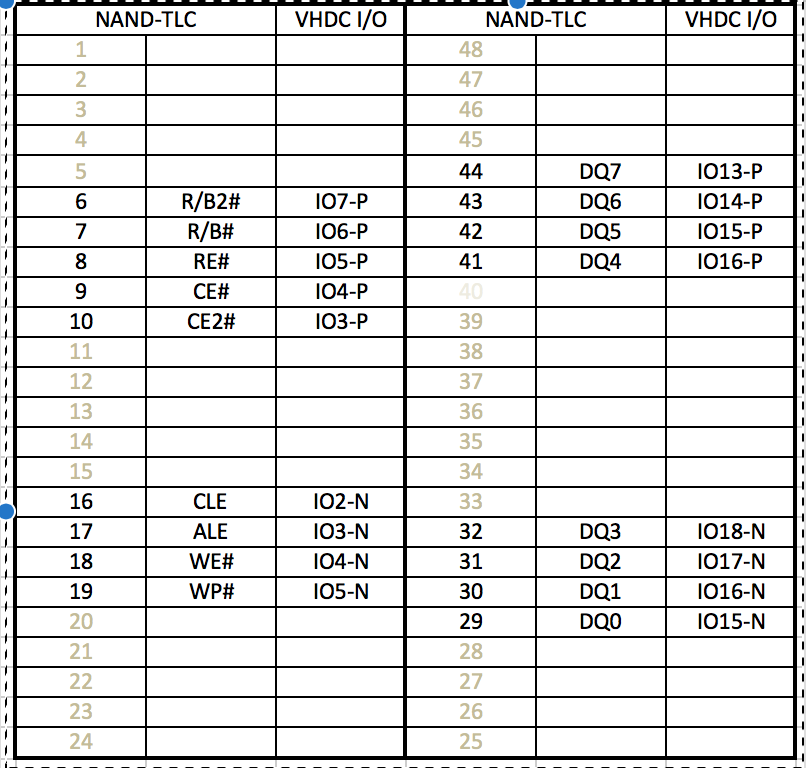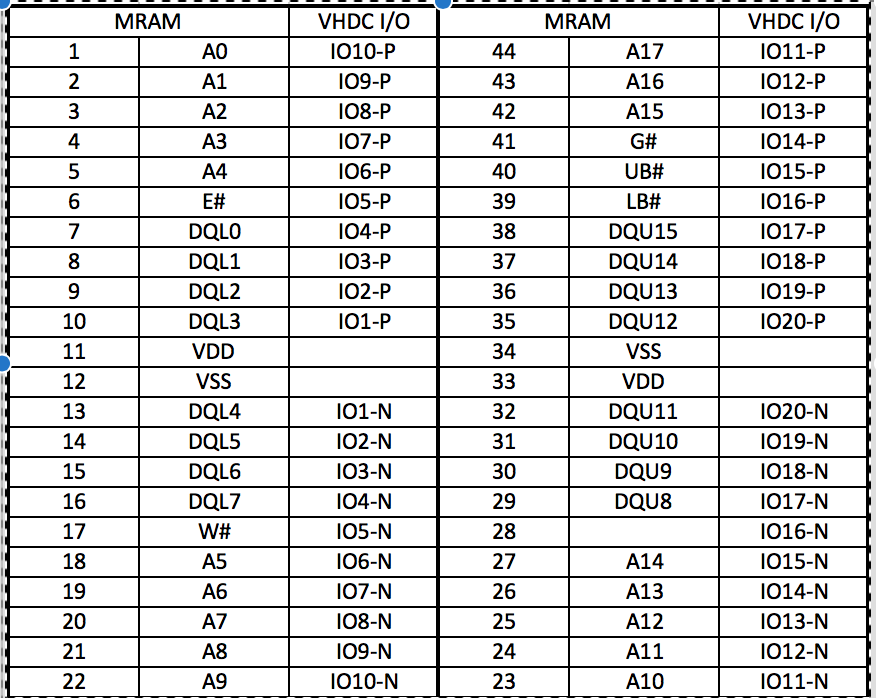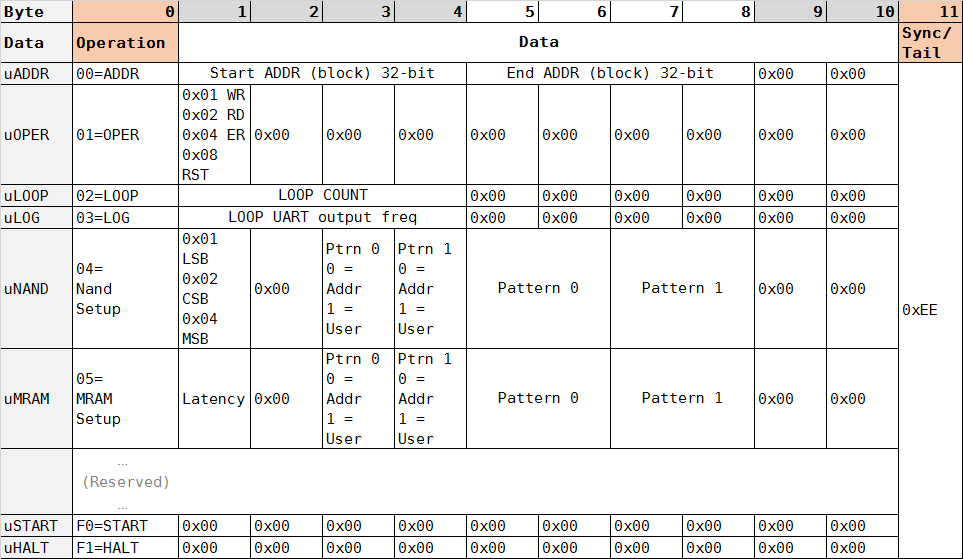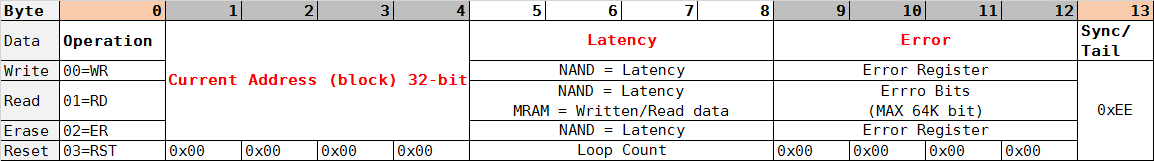# Code

1. State Machines

• Main Loop States
``````parameter
INIT_0     = 30, INIT_1     = 29, INIT_2     = 28,
RS_0       = 06, RS_1       = 07, RS_2       = 08, //RS_3       = 09,
WR_0       = 09, WR_1       = 10, //WR_2       = 12,
RD_0       = 11, RD_1       = 12, //RD_2       = 15,
ER_0       = 13, ER_1       = 14, //ER_2       = 18,
UART_0     = 15, UART_1     = 16, UART_2     = 17, UART_3     = 18,
INIT_FULL_LOOP = 19,
LOOP_END   = 31;
``````
• NVM-specific Loop States
``````parameter
INIT       = 30, INIT_T     = 31, STOP       = 00,
CMDA_0     = 01, CMDA_1     = 02, CMDA_2     = 03,
CMDB_0     = 08, CMDB_1     = 09, CMDB_2     = 10,
WAIT_CYL   = 11,
WAIT_RB_0  = 12, WAIT_RB_1  = 13,
DATA_IN_0  = 14, DATA_IN_1  = 15, DATA_IN_2  = 16, DATA_IN_3  = 17, DATA_IN_4  = 18,  //WRITE
DATA_OUT_0 = 19, DATA_OUT_1 = 20, DATA_OUT_2 = 21, DATA_OUT_3 = 22, DATA_OUT_4 = 23, DATA_OUT_5 = 24,  //READ
RESET_0    = 25, RESET_1    = 26, RESET_2    = 27, RESET_3    = 28, RESET_4    = 29;
``````
• Serial Loop
• TX
• RX
• Debug Loop
• There is a state machine for displaying all of 4 digits of 7-Segments
• ( FYI, LEDs are not in loop, just shows state number of NVM state machine. )
2. Physical pin definition

• In Verilog code, .ucf file defines which pins are connected to which pin.
• PIN names are described in Nexys 3 manual.
• Regarding pinout of PCB design, simply matching the pins definition on .ucf file makes it connection.

• TLC NAND pin-connection• MRAM pin-connection# Packet Structure

1. Common characteristics

• Baud-rate: 1152000 bps
• Using Big-Endian for data values

• In-code pySerial initialization

``````self.sp = serial.Serial(com_port_num, 115200, timeout=3)
``````
• In-code Verilog timer definition

```````define BIT_TMR_MAX 10'd869
``````
• How to calculate the counter number 869:
• Current FPGA Clock = 100Mhz ( 1tick = 10ns ), target baud-rate = 1152000bps
• (100,000,000ticks/s) / (115200bits/s) = 869ticks/bit
2. PC → Board (FPGA - RX)

• Configuration
• Start/Stop
• 12 bytes per each packet
• RAW Packet Structure• In-code implementation (Python part)
``````def uADDR(addr1, addr2):

def uOPER(oWR, oRD, oER, oRST):
mOPER = 0x0
if oWR : mOPER |= 0x01
if oRD : mOPER |= 0x02
if oER : mOPER |= 0x04
if oRST: mOPER |= 0x08
return struct.pack('>BBBBBIHB', 0x01, mOPER,0,0,0, 0, 0, 0xEE)

def uLOOP(loop_count):
return struct.pack('>BIIHB', 0x02, loop_count, 0, 0, 0xEE)

def uLOG(log_freq2):
return struct.pack('>BIIHB', 0x03, log_freq2, 0, 0, 0xEE)

def uNAND(pLSB, pCSB, pMSB, ptrn_usr0=0, ptrn_usr1=0, ptrn0=0, ptrn1=0xFF):
mPAGETYPE = 0
if pLSB : mPAGETYPE |= 0x01
if pCSB : mPAGETYPE |= 0x02
if pMSB : mPAGETYPE |= 0x04
return struct.pack('>BBBBBHHHB', 0x04, mPAGETYPE, 0, ptrn_usr0,ptrn_usr1, ptrn0,ptrn1, 0, 0xEE)

def uSTART():
return struct.pack('>BIIHB', 0xF0, 0, 0, 0, 0xEE)

def uHALT():
return struct.pack('>BIIHB', 0xF1, 0, 0, 0, 0xEE)
``````
3. Board → PC (FPGA - TX)

• Result
• 14 bytes per each packet
• This data is captured/saved into log file and processed with parser.
• RAW Packet Structure• In-code implementation (Verilog part)
``````assign tx_data[7:0]=(           (tx_idx ==  0) ? { 6'h0, Oper[1:0] } :                  //Oper
(          (tx_idx ==  1) ? { 8'h0 } :                             //ADDR
(      (tx_idx ==  5) ? { latency[31:24] } :
(     (tx_idx ==  6) ? { latency[23:16] } :
(    (tx_idx ==  7) ? { latency[15: 8] } :
(   (tx_idx ==  8) ? { latency[ 7: 0] } :
(  (tx_idx ==  9) ? { badbits_wire[31:24] } :
( (tx_idx == 10) ? { badbits_wire[23:16] } :
(tx_idx == 11) ? { badbits_wire[15: 8] } :
(tx_idx == 12) ? { badbits_wire[ 7: 0] } :
{ 8'hEE }
)
)
)
)
)
)
)
)
)
)
);
``````